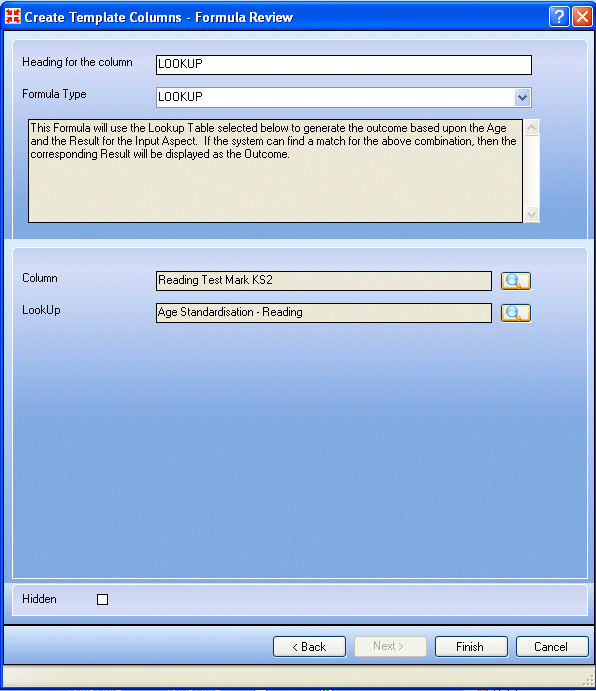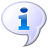# Getting Started In This Chapter

 Page 70/209 Date 08.07.2018 Size 12.14 Mb.

### Inserting a Max Formula

This formula is only available for use where the outcome of the calculation is stored in a Marks (Integer) or Marks (Decimal) aspect.

The formula will calculate the result with the maximum value in a set of selected columns. Alternatively, it can be used to return the result for any other position in the set of columns, e.g. 2nd, 3rd, 4th etc.

1. Select Max from the Formula Type drop-down list.

2. Choose the required columns (please see Choosing a Selection of Columns on page 143).

3. Enter the required number in the Max field. For example, if you have selected eight columns then entering 1 will return the maximum value from those columns, 2 will return the second highest value, and so on; whilst entering 8 will return the lowest value.
1. ### Inserting a Mean Formula

This formula is only available for use where the outcome of the calculation is stored in a grade, Marks (Integer), Marks (Decimal) or Comments aspect.

This formula will calculate the mean of the values found in the columns you select.

1. Select Mean from the Formula Type drop-down list.

2. Choose a selection of the columns to be averaged (please see Choosing a Selection of Columns on page 143).

NOTE: Where reference is made to an empty cell in a marksheet, the result is totally excluded from the calculation. Therefore, the mean of 3 + 3 + empty is 3. Where reference is made to cells displaying ages, the contents of the cell are converted to months before being included in the calculation. Where a reference is made to a cell displaying a grade, the value of the displayed grade is used.
1. ### Inserting a Value Formula

This formula is only available for use where the outcome of the calculation is stored in a Marks (Integer), Marks (Decimal) or Comments aspect.

The formula stores as the outcome, the Value of the grade found in the selected column (which must be a grade aspect). The Value is that defined in the grade set associated with the selected aspect.

1. Select Value from the Formula Type drop-down list.

2. Choose the required column by clicking the Field Browser button to display the Template Columns dialog.

3. Click the required column name then click the Select button.

NOTE: Where reference is made to an empty cell in a marksheet, a blank outcome is generated.
1. ### Inserting a Position Formula

This formula is only available for use where the outcome of the calculation is stored in a Marks (Integer), Marks (Decimal) or Comments aspect.

This formula will calculate the position of the pupil/student using the result found in the selected column, e.g. 1st, 5th, 10th, etc. Only Age, Marks or grade aspects can be selected.

1. Select Position from the Formula Type drop-down list.

2. Choose the required column by clicking the Field Browser button to display the Template Columns dialog.

3. Click the required column name then click the Select button.
1. ### Inserting an Age at Result Formula

This formula is only available for use where the outcome of the calculation is stored in an Age aspect.

This formula calculates the age of the pupil/students on the date they attained the result in the selected column.

1. Select Age At Result from the Formula Type drop-down list.

2. Choose the required column by clicking the Field Browser button to display the Template Columns dialog.

3. Click the required column name then click the Select button.

NOTE: This formula uses the result date (as selected on the marksheet in the Result Date field), not the date upon which the result was physically entered. Where reference is made to an empty cell in a marksheet, a blank outcome is generated.
1. ### Inserting a Current Age Formula

This formula is only available for use where the outcome of the calculation is stored in an Age aspect.

This formula calculates the age of the pupil/students on the selected date.

1. Select Current Age from the Formula Type drop-down list.

2. Select the required date by clicking the drop-down arrow to display the Calendar, which defaults to the current month.

3. Click the left or right arrows to view previous and future months respectively.

4. Once the required month has been found, select the required date to select it.

Alternatively, enter the date in dd/mm/yy format in the Date field.
1. ### Inserting a Percent Formula

This formula is only available for use where the outcome of the calculation is stored in a Marks (Integer), Marks (Decimal) or Comments aspect.

Calculates the value of the result found in the selected column as a percentage of the maximum value in the aspect definition. For example, aspect A has a minimum value of 0 and a maximum value of 50. When calculating this formula, the result is expressed as a percentage of 50.

1. Select Percentage from the Formula Type drop-down list.

2. Choose the required column by clicking the Field Browser button to display the Template Columns dialog.

3. Click the required column name then click the Select button.

NOTE: Where reference is made to an empty cell in a marksheet, a blank outcome is generated.
1. ### Inserting a Lookup Formula

The Lookup formula is for use with the Qualifications and Curriculum Authority (QCA) Age Standardised Scores and enables you to look at a pupil/student’s marks or grade and measure it against the national average for their age. Age standardised scores use a mean of 100.

Standardised scores take into account a child’s age in years and months, giving an indication of how each child is performing relative to other children of the same age. Age standardised scores do not affect the child’s test levels.

To use the Lookup formula, you first need to create the Lookup Table from the QCA data provided. You can then define the template, to include a Data Entry column that contains the Lookup formula, and then a marksheet to enter, calculate and display the results.

When defining a Lookup formula in a template it is possible to set an effective date to be used when the formula is calculated (instead of the system date, which is the default), That date is then stored with the result.

There can be more than one Lookup formula in a template and each can have a different effective date.

To insert the Lookup formula:

1. Select Lookup from the Formula Type drop-down list.1. Choose the column to be compared, by clicking the Field Browser button to display the Template Columns dialog.

2. Click the required column name then click the Select button.

3. Choose the Lookup Table to use, by clicking the Field Browser button to display the Lookup Browser dialog.

4. Click the required Lookup Table name then click the Select button.

5. To define an effective date to be used when the formula is calculated, enter the required dates in the From and To fields or click the appropriate drop-down arrow to select the required date from the calendar.

NOTE: You can only specify an Effective Date if the Lookup formula is being defined for a Data Review column.

1. Click the Finish button to return to the Template Details page.More Information: Creating Lookup Tables on page 105 Entering and Reviewing Data using Marksheets on page 5